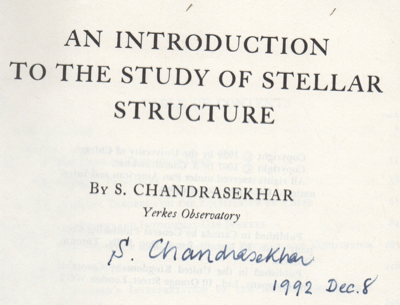Cococubed.com Polytropic Stars

Home

Astronomy research
Software instruments
Stellar equation of states
EOS with ionization
EOS for supernovae
Chemical potentials
Stellar atmospheres

Voigt Function
Jeans escape
Polytropic stars
Cold white dwarfs

Cold neutron stars
Stellar opacities
Neutrino energy loss rates
Ephemeris routines
Fermi-Dirac functions

Polyhedra volume
Plane - cube intersection
Coating an ellipsoid

Nuclear reaction networks
Nuclear statistical equilibrium
Laminar deflagrations
CJ detonations
ZND detonations

Fitting to conic sections
Unusual linear algebra
Derivatives on uneven grids

Supernova light curves
Exact Riemann solutions
1D PPM hydrodynamics
Hydrodynamic test cases
Galactic chemical evolution

Universal two-body problem
Circular and elliptical 3 body
The pendulum
Phyllotaxis

MESA
MESA-Web
FLASH

Zingale's software
Brown's dStar
GR1D code
Herwig's NuGRID
Meyer's NetNuc
Presentations
Illustrations
Public Outreach
Education materials
2022 ASU Solar Systems Astronomy
2022 ASU Energy in Everyday Life

AAS Journals
2022 Earendel, A Highly Magnified Star
2022 TV Columbae, Micronova
2022 White Dwarfs and 12C(α,γ)16O
2022 MESA in Don't Look Up
2022 MESA Marketplace
2022 MESA Summer School
2022 MESA Classroom
2021 Bill Paxton, Tinsley Prize

Contact: F.X.Timmes
my one page vitae,
full vitae,
research statement, and
teaching statement.

The code public_poly.tbz computes the structure of stars that are in hydrostatic equilibrium and obey a polytropic equation of state \begin{equation} P = K \rho^{\gamma} = K \rho^{1 + 1/n} \ . \label{eq1} \tag{1} \end{equation} The solution to the resulting Lane-Emden equation \begin{equation} \dfrac{d^2y}{dx^2} + \dfrac{2}{x} \dfrac{dy}{dx} + y^n = 0 \hskip 0.5in y(x=0)=1 \hskip 0.5in \left . \dfrac{dy}{dx}\right |_{x=0} = 0 \ , \label{eq2} \tag{2} \end{equation} where $x$ is a dimensionless radius and $y$ is a dimensionless density, is writen out in dimensionless form and in physical units. Certain polytropic stars are related cold white dwarfs.Pathways in the PV-plane for a polytropic equation of state. Note γ ≡ 1 + 1/n = n for the golden ratio of n = Φ = 1.6180…Numerical solutions to the Lane-Emden equation. For n < 5, the solutions can be continued to negative y. Although not physical, such solutions exists mathematically, and are quite useful for determining the values of x and dy/dx when y is zero. It's an exercise for the user to compare the computed surface values with other's tabulated values.Difference between the analytical and numerical solutions for n = 0, 1, and 5 for various integration accuracies.The larger n, the more extended the object. Other physical properties can be gleaned from the output files.It was a good day. Chicago. 2nd floor LASR. One in an impeccable brown suit and the other in blue overalls, white t-shirt, and Sear's DieHard steel-toe black shoes.

Please cite the relevant references if you publish a piece of work that use these codes, pieces of these codes, or modified versions of them. Offer co-authorship as appropriate.